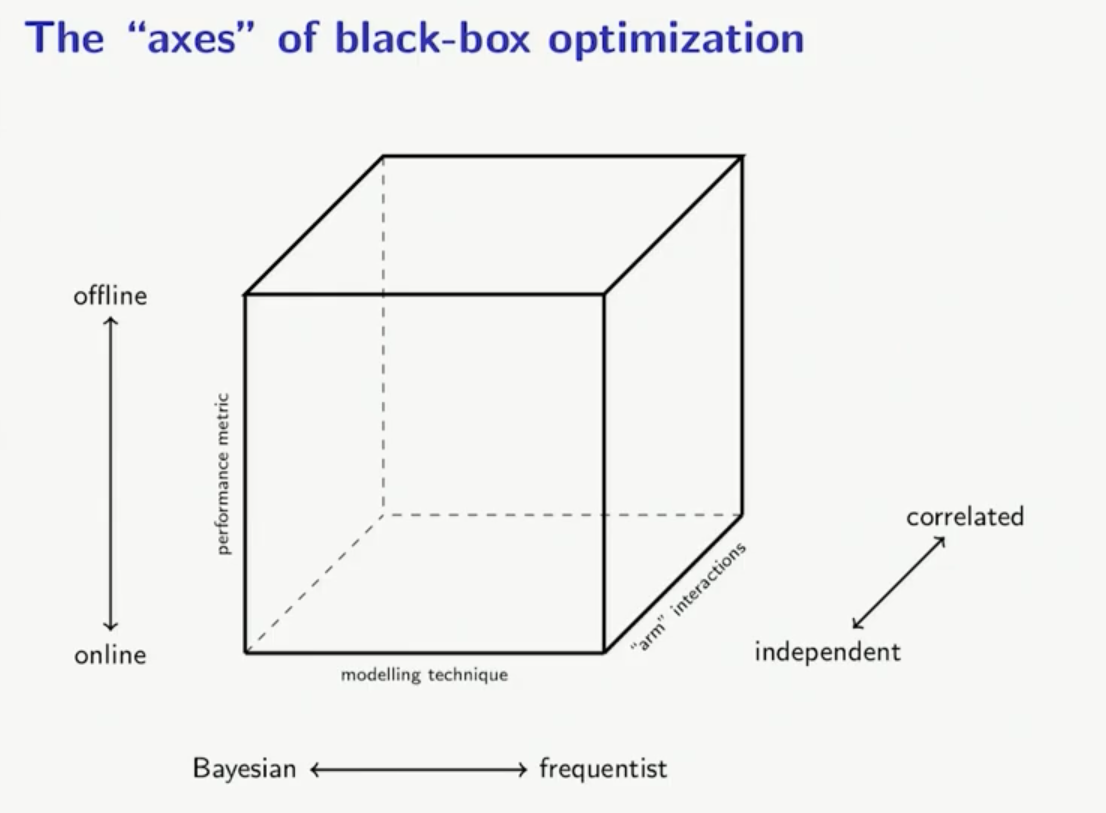# Bayesian Optimization §

First encountered this in the BayesRace paper.

Ahh it’s actually a great talk. There is this close parallel with Multi-Armed Bandit problems.

They have the same Exploration and Exploitation problem.

When you sample points that are bad, but you just want to confirm that they are bad.Two questions:

• What is my model?
• What is the exploration strategy?

Bayesian optimization is a sequential design strategy for global optimization of black-box functions that does not assume any functional forms. It is usually employed to optimize expensive-to-evaluate functions.

Goal: maximize subject to , where is some unknown function

BayesOpt is known for data-efficiency and is widely used in diverse applications such as:

• tuning hyperparameters of complex deep neural networks
• learning data-efficient reinforcement learning (RL) policies for robotic manipulation tasks
• tuning controller parameters in robotics
• optimal experiment design for designing functional tests in buildings
• recommender systems

Resources:

• There are so many parallels with this being a bandit problem. They talk Thompson Sampling